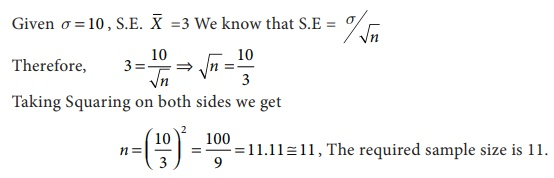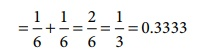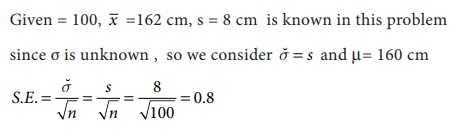Home | | Business Maths 12th Std | Computing standard error in simple cases

# Computing standard error in simple cases

Sampling and Non-Sampling Errors: Computing standard error in simple cases - Example Solved Problems with Answer, Solution, Formula

Computing standard error in simple cases

Example 8.6

A server channel monitored for an hour was found to have an estimated mean of 20 transactions transmitted per minute. The variance is known to be 4. Find the standard error.

Solution:Example 8.7

Find the sample size for the given standard deviation 10 and the standard error with respect of sample mean is 3.

Solution:Example 8.8

A die is thrown 9000 times and a throw of 3 or 4 is observed 3240 times. Find the standard error of the proportion for an unbiased die .

Solution:

If the occurrence of 3 or 4 on the die is called a success, then

Sample size = 9000; Number of Success = 3240

Sample proportion = p = 3240/9000 = 0 36.

Population proportion (P) = Prob(getting 3 or 4 when a die is thrown)Thus P = 0.3333 and Q = 1–P=1– 0.3333 = 0.6667

The S.E for sample proportion is given byHence the standard error for sample proportion is S.E=0.00496.

Example 8.9

The standard deviation of a sample of size 50 is 6.3. Determine the standard error whose population standard deviation is 6?

Solution:Example 8.10

A sample of 100 students is chosen from a large group of students. The average height of these students is 162 cm and standard deviation (S.D) is 8 cm. Obtain the standard error for the average height of large group of students of 160 cm?

Solution:Therefore the standard error for the average height of large group of students of 160 cm is 0.8.

Tags : Example Solved Problems with Answer, Solution | Sampling and Non-Sampling Errors , 12th Business Maths and Statistics : Chapter 8 : Sampling Techniques and Statistical Inference
Study Material, Lecturing Notes, Assignment, Reference, Wiki description explanation, brief detail
12th Business Maths and Statistics : Chapter 8 : Sampling Techniques and Statistical Inference : Computing standard error in simple cases | Example Solved Problems with Answer, Solution | Sampling and Non-Sampling Errors